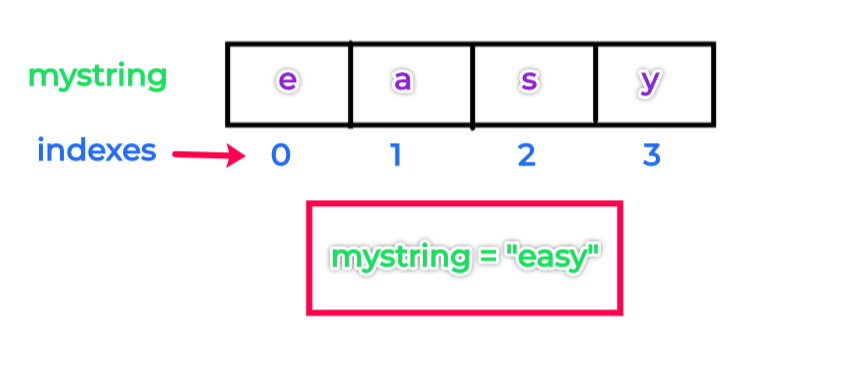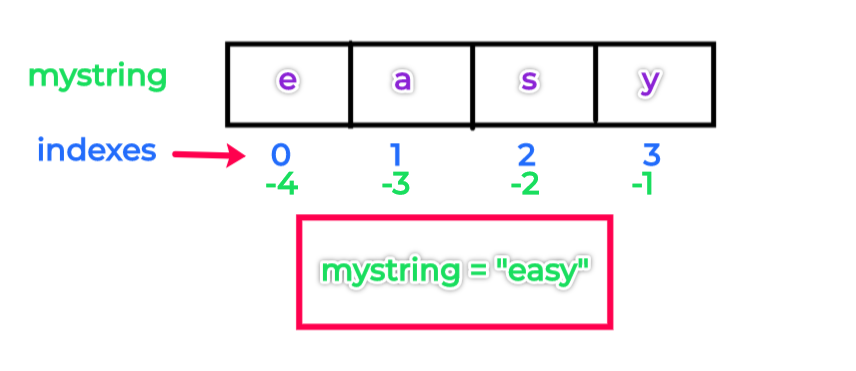# Python Strings

## Python String Datatype

A string literal is simply a list of characters (textual data) in sequence surrounded by quotes.
A character can be anything like numbers, backslash or letters and can also have space.
For example,`"easy"`is a string.
It is a four character long with each characters in sequence `"e", "a", "s", "y"`
We can assign the same string to a variable as follows

``````mystring = "easy" # create a 4 character string objects and assign it to name "mystring"
``````

mystring is the variable name to which the string object is assigned.
There are fours ways you can declare a string literal (or create a string object) in Python i.e. single quotes ('), double quotes ("), triple double quotes (""") and triple single quotes (''')
Example:

``````string1 = 'It is a single quoted string'
string2 = "It is a double quoted string"
string3 = """It is a triple double quoted string"""
string4 = '''It is a triple single quoted string'''
multiline_string1 = """
This is a
sting in multiple lines
"""
multiline_string2 = '''
This is a
sting in multiple lines
'''
unicode_string = u'e\xc4sy'
print(type(string1))
print(type(unicode_string))
``````

<class 'str'>
<class 'str'>

type() - is a built-in function which returns the type to which the object belongs. Refer to https://docs.python.org/3.7/library/functions.html#type
multilinestring1 and multilinestring2 are multi-line string literals. We can write multiple line texts/string using triple double quotes (""") and triple single quotes (''')
unicodestring is unicode string literal.

## Indexing in Python String

The characters are stored in order or sequence in the memory with a particular position assigned to each of the character.
The positions or the offsets are known as index.
Example:

``````mystring = "easy" # create a 4 character string objects and assign it to name "mystring"
``````
When we assign the value "easy" to mystring the value get stored in memory as followsThe first item or character "e" is at index 0 and the second item "a" is at index 1 ans so on
Items or characters from the string can be retrieved by using the name ( e.g. mystring ) and these indexes. The process is called indexing

As "e" is at index 0 we can retrieve and print "e" using name mystring as follows

``````print(mystring)
``````

e
To retrieve "a" and others

``````print(mystring)
print(mystring)
print(mystring)
``````

a
s
y

## Negative Index

we can also index backward. The above positive indexes from 0, 1, 2 and so on -count form left to right
The negative index count from right to left starting with -1 e.g. -1, -2, -3.
So from the previous example `mystring = "easy"`,if we want to retrieve "a" using mystring and negative index we can write

``````print(mystring[-3])  # is same as mystring
``````

a So the value at 1 is same as -3 and 0 is as index -4

## Slicing

We can also extract a portion of a string using indexes know as slicing.
The syntax is

``````variable_name[I:J] # Gives everything in variable_name from I to J, but inot including J
``````

Example:

``````print(mystring[1:3])
``````

as
So it extracts the string from offset/index 1 to 2 (Not including 3)

## Python String Operators

Python has a set of built-in methods that you can use on strings
"+" operator concatenates two strings on both sides of the operator Example:
``````>>> string1 = "py"
>>> string2 = "thon"
>>> string1 + string2
'python'``````
Concatenates and repeats string and creates new string Example:
``````>>> str_1 = "Python"
>>> str_1 * 3
'PythonPythonPython'``````
This operator suppresses the actual meaning of escape characters
Example:
``````>>> print('thi \nis ')
thi
is
>>> print(r'thi \nis ')
thi \nis
>>> print(R'thi \nis ')
thi \nis``````
In the above example 'R' or 'r' suppresses the meaning of '\n'
Without the raw string operator, the 'is' printed in the next line
Returns True if substring is present in whole string
Example:
``````>>> str_1 = "Python"
>>> "th" in str_1
True``````
Returns True if substring is NOT present in whole string
Example:
``````>>> str_1 = "Python"
>>> "a" not in str_1
True
>>> "on" not in str_1
False``````
This operator helps in formatting and replacing different datatype within it
Refer to - https://docs.python.org/2/library/stdtypes.html#string-formatting
Example:
``````>>> str_1 = "Python %s easy" % ('is')
>>> str_1
'Python is easy'
>>> str_2 = 'Today is %dth Nov' % 14
>>> str_2
'Today is 14th Nov'``````
Gives a character at the particular index
Example:
``````>>> str_1 = "Python"
>>> str_1
't'``````
Gives a sub string with a given range. Syntax: [startindex:endindex]
Example:
``````>>> str_1 = "Python"
>>> str_1[2:4]
'th'``````

## Python String methods

Python has a set of built-in methods that you can use on strings
Example:
``````>> string1 = "pythoneasy is python website"
>> string1.count('python')
2
```
```
Example:
``````>> string1 = "pythoneasy is python website"
>> string1.find('on')
4```
```
"on" substring is at index 4 and 19. But it prinsts the first index occurrence
Example:
``````>>> string1 = "python is easy"
>>> string1.upper()
'PYTHON IS EASY'```
```
Example:
``````>>> string1 = "PYTHON IS EASY"
>>> string1.lower()
'python is easy'```
```
Example:
``````>>> string1 = "Python Is Easy"
>>> string1.swapcase()
'pYTHON iS eASY'```
```
Returns a titlecased version of the string where words start with an uppercase character and the remaining characters are lowercase.
Example:
``````>>> string1 = "python is easy"
>>> string1.title()
'Python Is Easy'```
```
Example:
``````>>> phrase = 'lexical semantics'
>>> phrase.capitalize()
'Lexical semantics'``````
Example:
``````>>> string1 = "      python is easy          "
>>> string1
'      python is easy          '
>>> string1.strip()
'python is easy'``````
Example:
``````>>> list_1 = ['python', 'is', 'easy']
>>> ''.join(list_1)
'pythoniseasy'
>>> ' '.join(list_1)
'python is easy'
>>> print('\n'.join(list_1))
python
is
easy
>>> print('\t'.join(list_1))
python  is      easy``````
Example:
``````>>> string_1 = ' 1  2   3  '
>>> string_1.split()
['1', '2', '3']
>>> string_1 = ' 1 in 2  in  3  '
>>> string_1.split('in')
[' 1 ', ' 2  ', '  3  ']``````